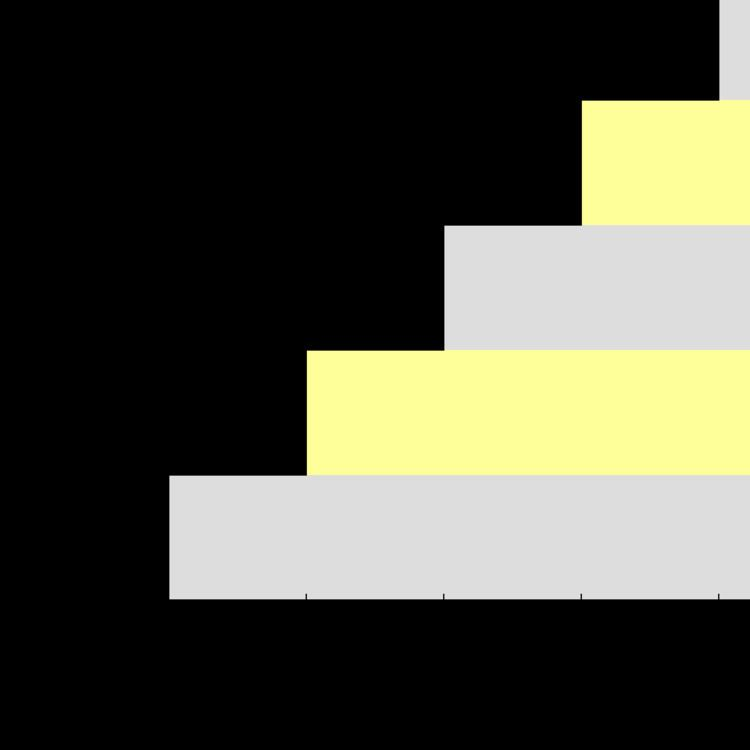# 1 1 1 1 ⋯

Updated on
Edit
Like
CommentIn mathematics, 1 + 1 + 1 + 1 + ⋯, also written n = 1 n 0 , n = 1 1 n , or simply n = 1 1 , is a divergent series, meaning that its sequence of partial sums does not converge to a limit in the real numbers. The sequence 1n can be thought of as a geometric series with the common ratio 1. Unlike other geometric series with rational ratio (except −1), it converges in neither the real numbers nor in the p-adic numbers for some p. In the context of the extended real number line

n = 1 1 = + ,

since its sequence of partial sums increases monotonically without bound.

Where the sum of n0 occurs in physical applications, it may sometimes be interpreted by zeta function regularization, as the value at s = 0 of the Riemann zeta function

ζ ( s ) = n = 1 1 n s = 1 1 2 1 s n = 1 ( 1 ) n + 1 n s ,

The two formulas given above are not valid at zero however, so one might try the analytic continuation of the Riemann zeta function,

ζ ( s ) = 2 s π s 1   sin ( π s 2 )   Γ ( 1 s )   ζ ( 1 s ) ,

Using this one gets (given that Γ(1) = 1),

ζ ( 0 ) = 1 π lim s 0   sin ( π s 2 )   ζ ( 1 s ) = 1 π lim s 0   ( π s 2 π 3 s 3 48 + . . . )   ( 1 s + . . . ) = 1 2

where the power series expansion for ζ(s) about s = 1 follows because ζ(s) has a simple pole of residue one there. In this sense 1 + 1 + 1 + 1 + ⋯ = ζ(0) = −1/2.

Emilio Elizalde presents an anecdote on attitudes toward the series:

In a short period of less than a year, two distinguished physicists, A. Slavnov and F. Yndurain, gave seminars in Barcelona, about different subjects. It was remarkable that, in both presentations, at some point the speaker addressed the audience with these words: 'As everybody knows, 1 + 1 + 1 + ⋯ = −1/2.' Implying maybe: If you do not know this, it is no use to continue listening.

1 + 1 + 1 + 1 + ⋯ Wikipedia